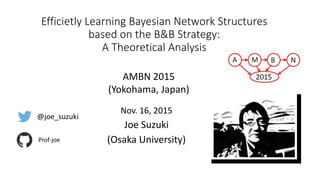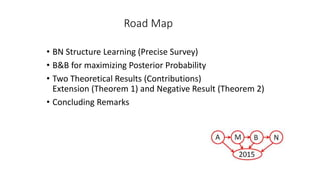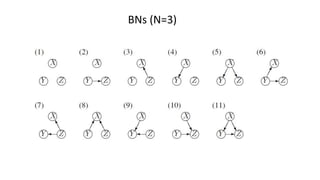Successfully reported this slideshow.

# Efficietly Learning Bayesian Network Structures based on the B&B Strategy: A Theoretical Analysis

1

Share×
1 of 22
1 of 22

# Efficietly Learning Bayesian Network Structures based on the B&B Strategy: A Theoretical Analysis

1

Share

Joe Suzuki, ``Efficietly Learning Bayesian Network Structures based on the B&B Strategy: A Theoretical Analysis", Yokohama, Japan, November 2016.

Joe Suzuki, ``Efficietly Learning Bayesian Network Structures based on the B&B Strategy: A Theoretical Analysis", Yokohama, Japan, November 2016.

## More Related Content

### Related Books

Free with a 14 day trial from Scribd

See all

### Related Audiobooks

Free with a 14 day trial from Scribd

See all

### Efficietly Learning Bayesian Network Structures based on the B&B Strategy: A Theoretical Analysis

1. 1. Efficietly Learning Bayesian Network Structures based on the B&B Strategy: A Theoretical Analysis Nov. 16, 2015 Joe Suzuki (Osaka University) AMBN 2015 (Yokohama, Japan) @joe_suzuki Prof-joe
2. 2. Road Map • BN Structure Learning (Precise Survey) • B&B for maximizing Posterior Probability • Two Theoretical Results (Contributions) Extension (Theorem 1) and Negative Result (Theorem 2) • Concluding Remarks
3. 3. BNs (N=3)
4. 4. BN Structure Learning (N=2)
5. 5. BN Structure Learning (N=3)
6. 6. proposed Dynamic Programming framework: • Finding the parent set of each variable • Ordering the variables to avoid making loops Silander-Milymaki (2006) Part I Part II
7. 7. MDL Jorma Rissanen
8. 8. MDL with B&B (Suzuki ICML‘96) Cut Rule Computing this and deeper can be saved
9. 9. An optimal parent set contains at most log n variables (Campos et.al, 2011)
10. 10. B&B Strategies for Maxmizing Posterior Probability for BDeu (Campos et. al., 2011)
11. 11. Problems 1. What is the exact formula for BD rather than BDeu? 2. How tight is the existing inequality? 3. At most how many variables the optimal parent set contains for maximizing the posterior rather than minimizing the description length?
12. 12. B&B Strategies for Maxmizing Posterior Probability for BD Theorem 1:
13. 13. A prior over parameters is regular less samples less biased
14. 14. Proof (outline) of Theorem 1 Beautiful derivation
15. 15. Main (negative) result for the cutting rule for BD/BDeu Theorem 2: No bound for how many variables the optinal π(X) contains (unlike log n for MDL)
16. 16. Proof (outline) of Theorem 2
17. 17. Concluding Remarks Learning BN with B&B for maximizing Posterior probability: • Cutting Rule for BD (extension of the existing bound, Theorem 1) • The obtained bound in Theorem 1 is so loose that no upper bound of the cardinality of optimal parent sets unlike MDL (Theorem 2) Future Work • Tighter Bound for Theorem 1